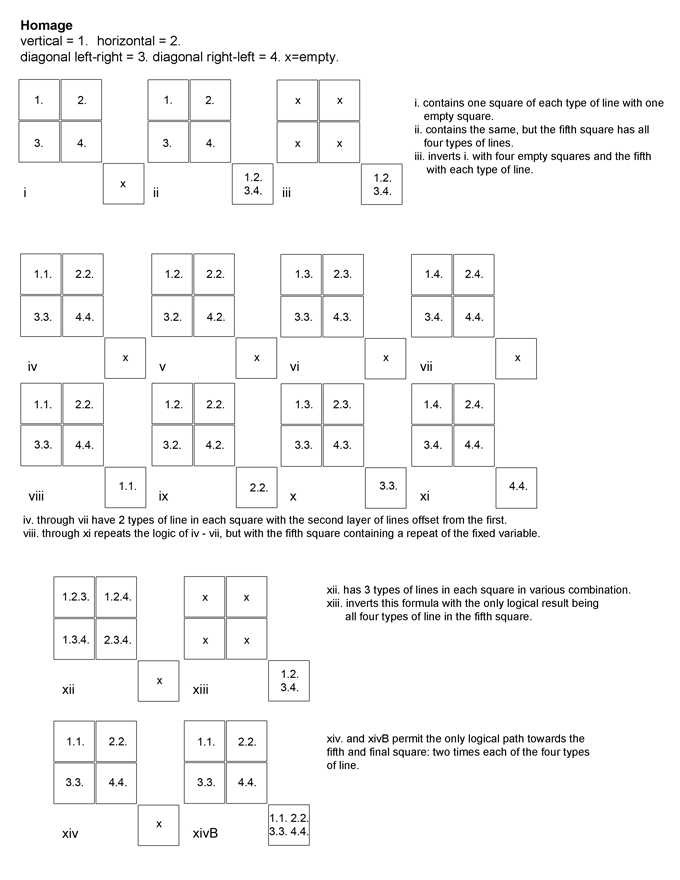### Homage & Spinoff | 2009 | digital drawings |

Homage is a set of 15 prints, numbered in roman from i - xivB. It is an homage to Sol LeWitt's many works using horizontal, vertical, and diagonal lines. Each print has 5 squares that contain vertical, horizontal, diagonal left to right, and diagonal right to left lines. Each print follows from the previous using a logical system of various combinations of these lines. A description of these 15 prints can be seen in the legend below. They are difficult to document as stills.

Spinoff is comprised of 4 sets of 10 prints which, using the same 5-square design as in Homage, variously combines vertical and horizontal lines with lines offset by 1-degree through 9-degrees. Two sets use vertical lines only. The first of these vertical line sets use layers of vertical [0-degree] and 1-degree lines, with each further degree of rotation layered atop in succession. The second of these vertical line sets begins with 1 layer of vertical lines, with each successive print adding a new layer of rotated lines so that the tenth piece has 10 layers superimposed, from 0 to 9 degrees.

The third and fourth sets of 10 follow the above formula, but in each case use both horizontal and vertical lines [a grid] as a base.